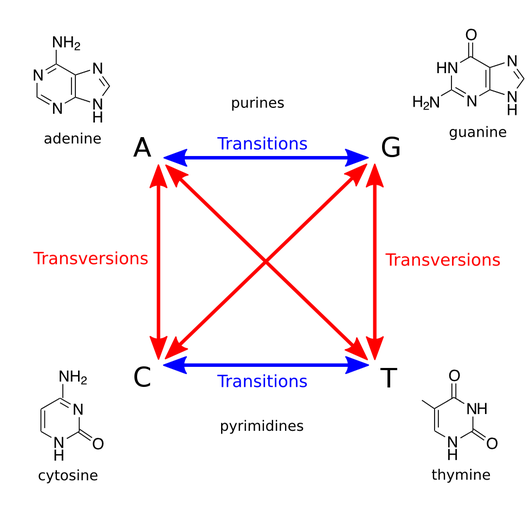# Glossary

## Transition/transversion ratio

Given two DNA or RNA strings $s_1$ and $s_2$ having the same length, their transition/transversion ratio $R(s_1, s_2)$ is the ratio of the number of transitions to the number of transversions (see the figure below), where symbol substitutions are inferred from those calculating Hamming distance.The transition/transversion ratio between homologous strands of DNA is generally about 2, but it is typically elevated in coding regions, where transversions are more likely to change the underlying amino acid and thus possibly lead to a fatal mutation in the translated protein. Point mutations that do not change this amino acid (which are thus more likely for transitions) are called silent substitutions.